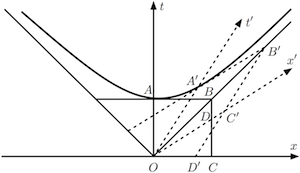# The Multispace Diagram vs Minkowski’s Diagram

In 1905, Einstein discovered the special relativity of inertial frames of reference, which generalizes Galileo’s principle of relativity (that all uniform motion is relative, and that there is no absolute and well-defined state of rest). He based his theory on the Lorentz transformation that describes how measurements of space and time in a moving frame of reference P’(x’, t’) depend on its motion relative to an observer in a stationary frame P(x, t).

Since then, the most famous contribution to Einstein’s theory of relativity belongs to Hermann Minkowski,1 who introduced the concept that space and time are fused in what he called the space-time continuum.2Figure 1. Minkowski diagram

To illustrate his concept, Minkowski built a two-dimensional space-time diagram (Figure 1), in which the space-time planes for two frames P and P’ are superposed.

During the Lorentz transformation, the coordinate axes x’ and t’ of the moving frame P’ rotate toward each other with respect to the corresponding axes x and t of the frame P at rest through an angle ψ that depends on the relative speed v between the two frames.

Over the last century, this simple yet intuitive visual aid helped clarify Einstein‘s theory, facilitating its dissemination to scientists and the general public.

Minkowski derived the four-dimensional formalism from his own relativity theory, but it was his heuristic two-dimensional diagram that worked magic in convincing the scientific world to adopt the spacetime continuum concept.

The Multispace Diagram (MSD) discovered by Eugeniu Alexandrescu is presented in the slideshow above, side by side with Minkowski’s diagram. MSD displays a 3D view of planes P and P’. Plane P’ rotates through an angle δ into dimension u.

Comparing the two views, we see that Minkowski’s diagram provides just a partial view of the Multispace Diagram. Clearly, his diagram represents the projection of the axes of plane P’ onto plane P. The relationship between angle ψ of Minkowski’s diagram and angle δ of MSD is given by the relationship:$\psi = \tan^{-1} \frac{1 - \cos \delta}{1 + \cos \delta}~.$

Observers in P cannot see, i.e. make direct measurements of events in P’. Even if they believe they have a direct view of P’, in reality they don’t. They can only see the projection of P’ on their own frame P.

In our daily life, when v<<c, observers don’t perceive any difference between really seeing things in P’ and seeing just their projection on P. The difference between the two reference frames becomes evident only when v is significantly higher: the higher the relative speed between the two frames, the larger the angle of this rotation and the smaller the projection of P’ on P.

Noteworthy, observers in P’ would see no reduction in the values of measurements of events in P’, no matter how fast P’ is moving with respect to P.

Until now, this unexpected and unknown dimension into which P’ rotates — let us coin it u, for “unknown” — has been hidden from our view and therefore missing from our understanding not just of special relativity, but of reality itself. Note that there are three u-dimensions, one for each spatial dimension.

We are accustomed to thinking of rotations in space. But the rotation of P’ away from P, although physical, does not depict a rotation into some spatial dimension. It is rather a relativistic rotation into u, which is related to the value of the relative speed between the two frames. So, we don’t even know if we could still call u a dimension according to the definition of this word in physics.

Another interpretation of u could be as a new degree of freedom for space-time frames themselves, rather than for the objects therein. Therefore, we must take extreme care when trying to grasp the subtle distinction between a relativistic rotation of one space-time frame of reference (relative to another frame) into dimension u and a physical rotation of an object within a frame of reference.

Furthermore, the fact that a frame of reference — in fact an independent space — can rotate relativistically within u suggests that space and time may not be the most fundamental elements of reality. Presently, the nature of dimension u is still a matter under inquiry.

The first hypothesis we can formulate is that:

To be able to rotate freely inside this extra dimension, the space-time of the moving frame must be different and separate from the space-time of the frame at rest. In other words, we cannot talk about just one space. There must be two independent physical spaces for relative motion to occur, and not, as Einstein thought, merely two abstract mathematical coordinate systems.

Consequently, we can formulate the hypothesis of the Multispace Model of the universe as follows:

Our universe is not just a unique space-time continuum as we believe, but a multispace holding countless real and independent spaces, which are inextricably tied to the energy and/or matter (in fact, other spaces) existing within them.

ENDNOTES

1 Hermann Minkowski was Einstein’s former mathematics teacher at the Federal Institute of Technology in Zürich.

2 In physics, spacetime (space-time, space time or space-time continuum) is any mathematical model that combines space and time into a single continuum. Spacetime is usually interpreted with space as being three-dimensional and time playing the role of a fourth dimension that is of a different sort than the spatial dimensions. Here, we use space-time in relation to the two space-time diagrams, which use just one dimension of space and time, the other two spatial dimensions not being affected by the Lorentz transformation.
Posted in Featured, Mathematics, News, Physics